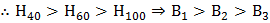# Three electric bulbs with same voltage ratings of 110 volts but wattage ratings of 40, 60 and 100 watts respectively are connected in series across a 220 volt supply line. If their brightness arerespectively, then a)b)c)d) Bulbs will burn out due to the high voltage supply

## Question ID - 150217 :- Three electric bulbs with same voltage ratings of 110 volts but wattage ratings of 40, 60 and 100 watts respectively are connected in series across a 220 volt supply line. If their brightness arerespectively, then a)b)c)d) Bulbs will burn out due to the high voltage supply

3537

 (a) Resistance of a bulbFor a given voltageRate of heat produced,When the bulbs are connected in series, the current flowing through each bulb is sameAsConsider a rectangular slab of lengthand area of cross sectionA currentis passed through it. If the length is doubled, the potential drop across the end faces a) Becomes half of the initial value b) Becomes one-fourth of the initial value c) Becomes double the initial value d) Remains same